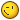# TargetCamera's rotation issue

## Recommended Posts

hi,

i'm working with FreeCamera, which is a TargetCamera.

if you move the mouse left or right, the camera rotates according to your movement, around y axis by default; but if you change the upVector, it rotates according the old configuration!

in my opinion, cameraRotation should be applied depending to the actual upVector, not the defaultUpVector, isn't right?

looking for usages of member upVector in TargetCamera, i see that it's updated only in _updateCameraRotationMatrix function and used only in _getViewMatrix.

furthermore, it's normalized in setTarget function, but it isn't used there (defaultUpVector is used in its place).

thanks

##### Share on other sites

this is the playground repro of the (presumed) issue.

thanks!

##### Share on other sites

this is the setTarget() method of the target camera. why i normalize the upVector if i don't use it (use defaultUpVector instead)

``````// Target
TargetCamera.prototype.setTarget = function (target) {
this.upVector.normalize();
BABYLON.Matrix.LookAtLHToRef(this.position, target, this._defaultUpVector, this._camMatrix);
this._camMatrix.invert();
this.rotation.x = Math.atan(this._camMatrix.m / this._camMatrix.m);
var vDir = target.subtract(this.position);
if (vDir.x >= 0.0) {
this.rotation.y = (-Math.atan(vDir.z / vDir.x) + Math.PI / 2.0);
}
else {
this.rotation.y = (-Math.atan(vDir.z / vDir.x) - Math.PI / 2.0);
}
this.rotation.z = 0;
if (isNaN(this.rotation.x)) {
this.rotation.x = 0;
}
if (isNaN(this.rotation.y)) {
this.rotation.y = 0;
}
if (isNaN(this.rotation.z)) {
this.rotation.z = 0;
}
if (this.rotationQuaternion) {
BABYLON.Quaternion.RotationYawPitchRollToRef(this.rotation.y, this.rotation.x, this.rotation.z, this.rotationQuaternion);
}
};``````

i would use the real upVector instead of the defaultUpVector.

running this function for an arbitrary upVector, it seems that the following code to recreate the camera orientation are wrong.

i would substitute the function with this version (slower but correct, need improvement):

``````// Target
TargetCamera.prototype.setTarget = function (target) {
this.upVector.normalize();
BABYLON.Matrix.LookAtLHToRef(this.position, target, this.upVector, this._camMatrix);
this._camMatrix.invert();

var scale = new BABYLON.Vector3();
var rotationQuat = new BABYLON.Quaternion();
var translation = new BABYLON.Vector3();
var decomposition = this._camMatrix.decompose(scale, rotationQuat, translation);
var rotation = rotationQuat.toEulerAngles();

this.rotation = rotation;

if (this.rotationQuaternion) {
BABYLON.Quaternion.RotationYawPitchRollToRef(this.rotation.y, this.rotation.x, this.rotation.z, this.rotationQuaternion);
}
};``````

now the setTarget function works for every upVector.

i'm still working to a fix for the camera movement... and i'd like to answer to some questions first:

1) what is this code for? i see everywhere those "if" snippets checking if rotationQuaternion is set to use it instead, but it appears that it is never used...

``````if (this.rotationQuaternion) {
BABYLON.Quaternion.RotationYawPitchRollToRef(this.rotation.y, this.rotation.x, this.rotation.z, this.rotationQuaternion);
}``````

2) here, only if using quaternion version, we update upVector. why?

``````//why to update upVector only if using quaternion?
TargetCamera.prototype._updateCameraRotationMatrix = function () {
if (this.rotationQuaternion) {
this.rotationQuaternion.toRotationMatrix(this._cameraRotationMatrix);
//update the up vector!
BABYLON.Vector3.TransformNormalToRef(this._defaultUpVector, this._cameraRotationMatrix, this.upVector);
}
else {
BABYLON.Matrix.RotationYawPitchRollToRef(this.rotation.y, this.rotation.x, this.rotation.z, this._cameraRotationMatrix);
}
};``````

``this.rotation.x = Math.atan(this._camMatrix.m / this._camMatrix.m);``

should be substituted by:

``this.rotation.x = Math.atan(this._camMatrix.m / this._camMatrix.m);``

or is there an hidden transposition i haven't noticed?

##### Share on other sites

So first of all we cannot use allocation in this method because it is called on every frame. (So no new Matrix/Vector3).

1. WebVR camera works with quaternion and not euler angles

2.Bug :0

3.Your article works with a different system (probably a transpose of our)

##### Share on other sites

Thanks, tomorrow I'll optimize the function and continue to work on camera movement## Join the conversation

You can post now and register later. If you have an account, sign in now to post with your account.
Note: Your post will require moderator approval before it will be visible.×   Pasted as rich text.   Paste as plain text instead

Only 75 emoji are allowed.